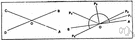plane angle

(redirected from Angle, Plane)
Also found in: Thesaurus, Encyclopedia.
Related to Angle, Plane: plane surface

plane angle

n.
An angle formed by two straight lines in the same plane.

plane angle

n
(Mathematics) an angle between two intersecting lines

plane′ an′gle

n.
an angle between two intersecting lines.
[1820–30]
ThesaurusAntonymsRelated WordsSynonymsLegend:
 Noun 1plane angle - an angle formed by two straight lines (in the same plane)angle - the space between two lines or planes that intersect; the inclination of one line to another; measured in degrees or radians
Site: Follow: Share:
Open / Close